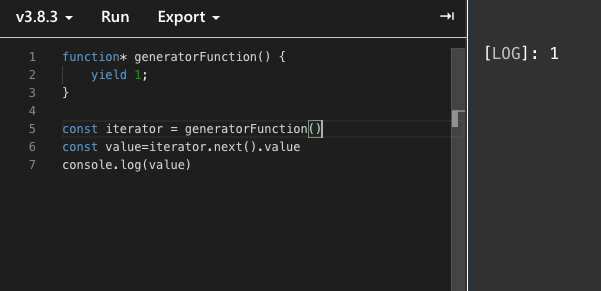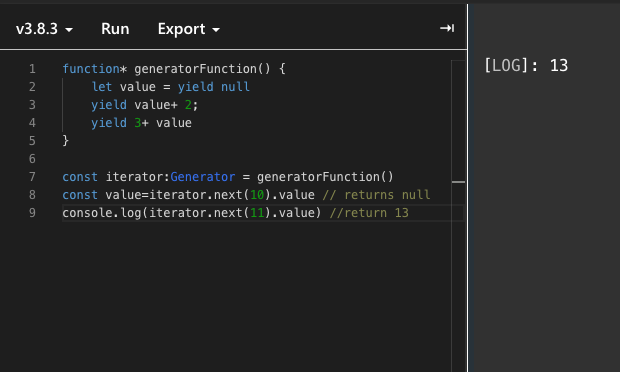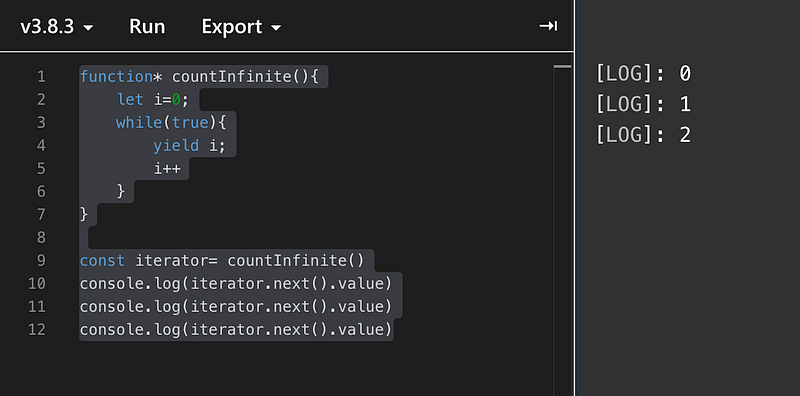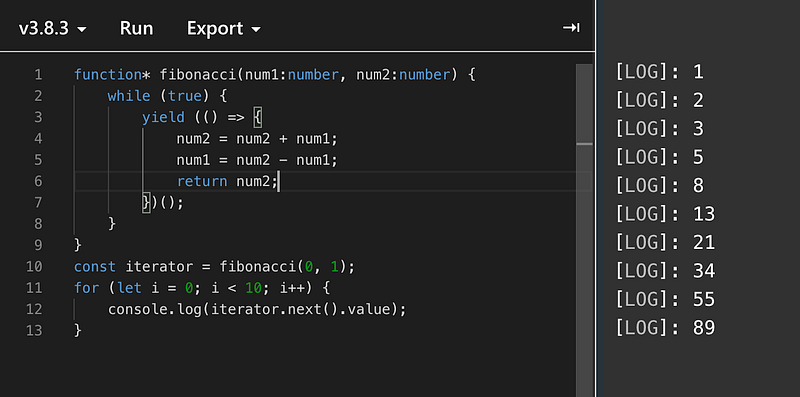With ES6, EcmaScript releases a new way of working with the functions. In this article we will take a look at them and how and where we can use them

What are the generator functions?

Generator functions are a special type of function which allows you to suspend their execution and later resumed at any time. Thye have also simplified the creation of iterators but we will get into that later. Let’s start simply by understanding what they are with some examples.

Creating a generator function is simple. The `function*` declaration (`function` keyword followed by an asterisk) defines a generator function.

``````function* generatorFunction() {
yield 1;
}
``````

Now, in generator functions, we don’t use return statements but rather a `yield` which specifies the value to be returned from the iterator. Now in the above example, it will return us a value of 1. Now, when we call generator functions like a normal ES6 function it does not directly execute the function but rather returns a `Generator` object. The `Generator` object contains `next()` , `return` and `throw` which can be used to interact with our generator functions. It works similarly to a `iterator` but you have more control over it.

Let’s see with an example of how we can use `generatorFunction` . Now, as I told you before we get `next()` . Now, the `next()` method returns an object with two properties `done` and `value`. You can also provide a parameter to the `next` method to send a value to the generator. Let’s see this with an example.

``````function* generatorFunction() {
yield 1;
}
const iterator = generatorFunction()
const value=iterator.next().value
console.log(value)
``````Now, as I said earlier that we can also pass values to the generator function through `next` and that value can be used inside `generator` the function. Let’s see how that works with another example.

``````function* generatorFunction() {
let value = yield null
yield value+ 2;
yield 3 + value
}
const iterator:Generator = generatorFunction()
const value=iterator.next(10).value // returns null
console.log(iterator.next(11).value) //return 13
``````Now, here when you obtain the generator you don’t have a yield you can push values to. So first you have to reach a yield by calling the next on the generator initially. It will return `null` always. You can pass arguments or not it does not matter it will always return `null` . Now, once you have done that you have an `yield` at your disposal and you can push your value via `iterator.next()` which will effectively replace `yield null` with the input passed through `next` and then when it finds another `yield` it returns back to the consumer of the generator which is our `iterator` here.

Now, let’s talk a little about the `yeild` keyword. Here, it looks like it’s working like return but on steroids because return simply returns a value from a function after a function is called and it will also not allow you to do anything after `return` keyword in a normal function but in our case `yield` is doing much more than that it’s returning a value but when you again call it, it will move on to the next `yield` statement. The `yield` keyword is used to pause and resume a generator function. The `yield` returns an object and it contains a `value` and `done` . The `value` is the result of the evaluating of the generator functions and the `done` indicates whether our generator function has been fully completed or not, its values can be either `true` or `false` . We can also use `return` keyword in generator function and it will return the same object but it will not go any further than that and the code after return will never be reached even if you have 6 `yield` after that so you need to be very careful using the `return` and should only be used once you are certain the job of the generator function is done.

``````function* generatorFunction() {
yield  2;
return 2;
yield 3; //generator function will never reach here
}
const iterator:Generator = generatorFunction()
``````

### Uses of Generator Function

Now, generator functions can very easily simplify the creation of iterators, implementation of the recursion or better async functionality. Let’s look at some examples.

``````function* countInfinite(){
let i=0;
while(true){
yield i;
i++
}
}
const iterator= countInfinite()
console.log(iterator.next().value)
console.log(iterator.next().value)
console.log(iterator.next().value)
``````In the above, it’s an infinite loop but it will only be executed as many times as we call `next` on the iterator and since it preserves the previous state of the function it continues to count. This is just a very basic example of how it can be used but we can use more complex logic inside the generator functions giving us more power.

``````function* fibonacci(num1:number, num2:number) {
while (true) {
yield (() => {
num2 = num2 + num1;
num1 = num2 - num1;
return num2;
})();
}
}
const iterator = fibonacci(0, 1);
for (let i = 0; i < 10; i++) {
console.log(iterator.next().value);
}
``````Now in the above example, we implemented a Fibonacci series without any recursion. The generator functions are really powerful and are only limited by your own imagination. Another big advantage of generator functions is that they are really memory efficient. We generate a value that is needed. In case of a normal function, we generate a lot of values without even knowing whether we are going to use them or not. However, in the case of the generator function, we can defer the computation and only use it when needed.

Now before using the generator function just keep some things in mind that you cannot access a value again if you have already accessed.

### Conclusion

Iterator functions are a great and efficient way to do a lot of things in javaScript. There are many other possible ways of using a generator function. For example, working with asynchronous operations can be made easy, now since a generator function can emit many values over time it can be used as an observable too. I hope this article helped you understand a little about `generator` function and let me know what else you can or are doing with the `generator` function.